# Nyquist Plot: What is it? (And How To Draw One)

## What is Nyquist Plot

A Nyquist plot (or Nyquist Diagram) is a frequency response plot used in control engineering and signal processing. Nyquist plots are commonly used to assess the stability of a system with feedback. In Cartesian coordinates, the real part of the transfer function is plotted on the X axis, and the imaginary part is plotted on the Y axis. The frequency is swept as a parameter, resulting in a plot based on frequency. The same Nyquist plot can be described using polar coordinates, where gain of the transfer function is the radial coordinate, and the phase of the transfer function is the corresponding angular coordinate.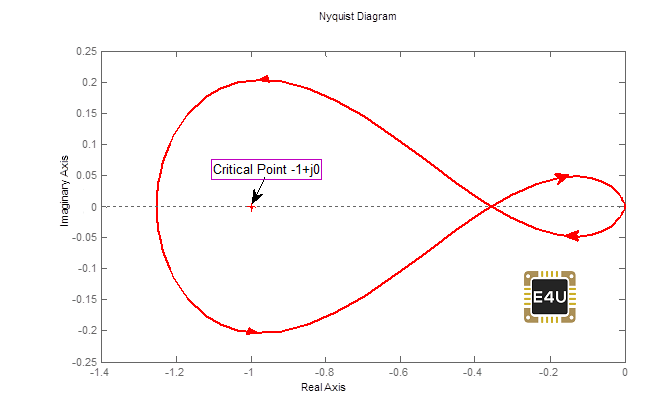The stability analysis of a feedback control system is based on identifying the location of the roots of the characteristic equation on s-plane. The system is stable if the roots lie on the left-hand side of s-plane. The relative stability of a system can be determined by using frequency response methods – such as the Nyquist plot and Bode plot.

The Nyquist stability criterion is used to identify the presence of roots of a characteristic equation in a specified region of s-plane. To understand a Nyquist plot we first need to learn about some of the terminologies. Note that a closed path in a complex plane is called contour.

## Nyquist Path or Nyquist Contour

The Nyquist contour is a closed contour in the s-plane which completely encloses the entire right-hand half of s-plane. In order to enclose the complete RHS of s-plane a large semicircle path is drawn with diameter along jω axis and center at the origin. The radius of the semicircle is treated as Nyquist Encirclement.

## Nyquist Encirclement

A point is said to be encircled by a contour if it is found inside the contour.

## Nyquist Mapping

The process by which a point in s-plane is transformed into a point in F(s) plane is called mapping and F(s) is called mapping function.

### How to Draw Nyquist Plot

A Nyquist plot can be drawn using the following steps:

• Step 1 – Check for the poles of G(s) H(s) of jω axis including that at origin.
• Step 2 – Select the proper Nyquist contour – a) Include the entire right half of s-plane by drawing a semicircle of radius R with R tends to infinity.
• Step 3 – Identify the various segments on the contour with reference to Nyquist path
• Step 4 – Perform the mapping segment by segment by substituting the equation for the respective segment in the mapping function. Basically, we have to sketch the polar plots of the respective segment.
• Step 5 – Mapping of the segments are usually mirror images of mapping of the respective path of +ve imaginary axis.
• Step 6 – The semicircular path which covers the right half of s plane generally maps into a point in G(s) H(s) plane.
• Step 7- Interconnect all the mapping of different segments to yield the required Nyquist diagram.
• Step 8 – Note the number of clockwise encirclement about (-1, 0) and decide stability by N = Z – P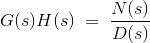is the Open loop transfer function (O.L.T.F)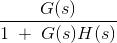is the Closed loop transfer function (C.L.T.F)
N(s) = 0 is the open loop zero and D(s) is the open loop pole
From stability point of view no closed loop poles should lie in the RH side of s-plane. Characteristics equation 1 + G(s) H(s) = 0 denotes closed loop poles .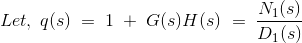Now as 1 + G(s) H(s) = 0 hence q(s) should also be zero.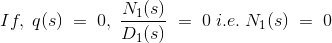Therefore, from the stability point of view zeroes of q(s) should not lie in RHP of s-plane.
To define the stability entire RHP (Right-Hand Plane) is considered. We assume a semicircle which encloses all points in the RHP by considering the radius of the semicircle R tends to infinity. [R → ∞].

The first step to understanding the application of Nyquist criterion in relation to the determination of stability of control systems is mapping from s-plane to G(s) H(s) – plane. s is considered as an independent complex variable and the corresponding value of G(s) H(s) being the dependent variable plotted in another complex plane called G(s) H(s) – plane.

Thus for every point in s-plane, there exists a corresponding point in G(s) H(s) – plane. During the process of mapping, the independent variable s is varied along a specified path in s – plane and the corresponding points in G(s)H(s) plane are joined. This completes the process of mapping from s-plane to G(s)H(s) – plane.

Nyquist stability criterion says that N = Z – P. Where, N is the total no. of encirclement about the origin, P is the total no. of poles and Z is the total no. of zeroes.
Case 1: N = 0 (no encirclement), so Z = P = 0 and Z = P
If N = 0, P must be zero therefore system is stable.
Case 2: N > 0 (clockwise encirclement), so P = 0, Z ≠0 and Z > P
For both cases system is unstable.
Case 3: N < 0 (counter clockwise encirclement), so Z = 0, P ≠0 and P > Z
System is stable.

Want To Learn Faster? 🎓
Get electrical articles delivered to your inbox every week.
No credit card required—it’s 100% free.